# Algebra II : Using e

## Example Questions

2 Next →

### Example Question #196 : Understanding Exponents

Simplify: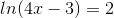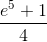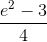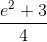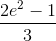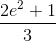Explanation:

In order to solve for the x-variable, we will need to raise both sides as powers of base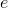, since the natural log has a default base of.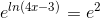The equation becomes: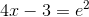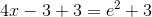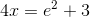Divide by four on both sides.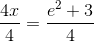The equation is: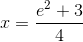The answer is:### Example Question #191 : Exponents

On the day of a child's birth, a sum of money is to be invested into a certificate of deposit (CD) that draws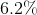annual interest compounded continuously. The plan is for the value of the CD to be at least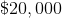on the child's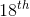birthday.

If the amount of money invested is to be a multiple of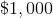, what is the minimum that should be invested initially, assuming that there are no further deposits or withdrawals?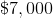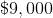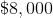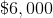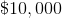Explanation:

If we letbe the initial amount invested andbe the annual interest rate of the CD expressed as a decimal, then at the end ofyears, the amount of moneythat the CD will be worth can be determined by the formula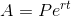Substitute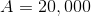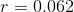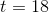, and solve for.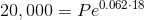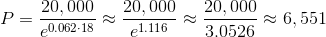The minimum principal to be invested initially is $6,551. However, since we are looking for the multiple of$1,000 that guarantees a minimum final balance of $20,000, we round up to the nearest such multiple, which is$7,000 - the correct response.

2 Next →

### All Algebra II Resources